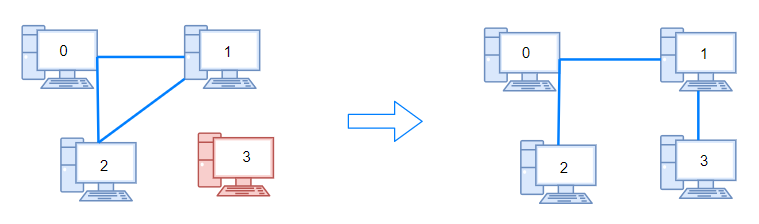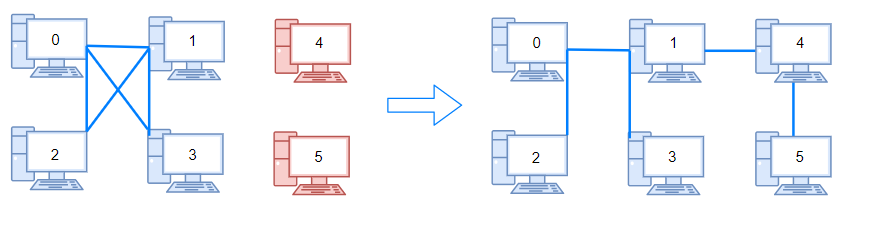##### Welcome to Subscribe On Youtube

Formatted question description: https://leetcode.ca/all/1319.html

# 1319. Number of Operations to Make Network Connected (Medium)

There are n computers numbered from 0 to n-1 connected by ethernet cables connections forming a network where connections[i] = [a, b] represents a connection between computers a and b. Any computer can reach any other computer directly or indirectly through the network.

Given an initial computer network connections. You can extract certain cables between two directly connected computers, and place them between any pair of disconnected computers to make them directly connected. Return the minimum number of times you need to do this in order to make all the computers connected. If it's not possible, return -1.

Example 1:Input: n = 4, connections = [[0,1],[0,2],[1,2]]
Output: 1
Explanation: Remove cable between computer 1 and 2 and place between computers 1 and 3.


Example 2:Input: n = 6, connections = [[0,1],[0,2],[0,3],[1,2],[1,3]]
Output: 2


Example 3:

Input: n = 6, connections = [[0,1],[0,2],[0,3],[1,2]]
Output: -1
Explanation: There are not enough cables.


Example 4:

Input: n = 5, connections = [[0,1],[0,2],[3,4],[2,3]]
Output: 0


Constraints:

• 1 <= n <= 10^5
• 1 <= connections.length <= min(n*(n-1)/2, 10^5)
• connections[i].length == 2
• 0 <= connections[i], connections[i] < n
• connections[i] != connections[i]
• There are no repeated connections.
• No two computers are connected by more than one cable.

Related Topics:
Depth-first Search, Breadth-first Search, Union Find

## Solution 1.

• class Solution {
final int WHITE = 0;
final int GRAY = 1;
final int BLACK = 2;

public int makeConnected(int n, int[][] connections) {
int length = connections.length;
if (length < n - 1)
return -1;
Map<Integer, List<Integer>> connectionsMap = new HashMap<Integer, List<Integer>>();
for (int[] connection : connections) {
int computer1 = connection, computer2 = connection;
List<Integer> list1 = connectionsMap.getOrDefault(computer1, new ArrayList<Integer>());
connectionsMap.put(computer1, list1);
List<Integer> list2 = connectionsMap.getOrDefault(computer2, new ArrayList<Integer>());
connectionsMap.put(computer2, list2);
}
int[] colors = new int[n];
int[] groups = new int[n];
for (int i = 0; i < n; i++)
groups[i] = i;
for (int i = 0; i < n; i++) {
if (colors[i] == WHITE)
}
Set<Integer> set = new HashSet<Integer>();
for (int i = 0; i < n; i++)
return set.size() - 1;
}

public void breadthFirstSearch(Map<Integer, List<Integer>> connectionsMap, int[] colors, int[] groups, int start) {
queue.offer(start);
colors[start] = GRAY;
while (!queue.isEmpty()) {
int computer = queue.poll();
int group = groups[computer];
List<Integer> list = connectionsMap.getOrDefault(computer, new ArrayList<Integer>());
for (int nextComputer : list) {
if (colors[nextComputer] == WHITE) {
queue.offer(nextComputer);
colors[nextComputer] = GRAY;
groups[nextComputer] = group;
}
}
colors[start] = BLACK;
}
}
}

############

class Solution {
private int[] p;

public int makeConnected(int n, int[][] connections) {
p = new int[n];
for (int i = 0; i < n; ++i) {
p[i] = i;
}
int cnt = 0;
for (int[] e : connections) {
int a = e;
int b = e;
if (find(a) == find(b)) {
++cnt;
} else {
p[find(a)] = find(b);
--n;
}
}
return n - 1 > cnt ? -1 : n - 1;
}

private int find(int x) {
if (p[x] != x) {
p[x] = find(p[x]);
}
return p[x];
}
}

• // OJ: https://leetcode.com/problems/number-of-operations-to-make-network-connected/
// Time: O(A * logN)
// Space: O(N)
class UnionFind {
vector<int> rank, id;
int cnt;
public:
UnionFind(int n): rank(n, 1), id(n), cnt(n) {
for (int i = 0; i < n; ++i) id[i] = i;
}
int find(int x) {
if (x == id[x]) return x;
return id[x] = find(id[x]);
}
void connect(int a, int b) {
int x = find(a), y = find(b);
if (x == y) return;
if (rank[x] <= rank[y]) {
id[x] = y;
if (rank[x] == rank[y]) rank[y]++;
} else id[y] = x;
--cnt;
}
int getCount() { return cnt; }
};
class Solution {
public:
int makeConnected(int n, vector<vector<int>>& A) {
if (A.size() < n - 1) return -1;
UnionFind uf(n);
for (auto &v : A) uf.connect(v, v);
return uf.getCount() - 1;
}
};

• class Solution:
def makeConnected(self, n: int, connections: List[List[int]]) -> int:
def find(x):
if p[x] != x:
p[x] = find(p[x])
return p[x]

cnt = 0
p = list(range(n))
for a, b in connections:
if find(a) == find(b):
cnt += 1
else:
p[find(a)] = find(b)
n -= 1
return -1 if n - 1 > cnt else n - 1


• func makeConnected(n int, connections [][]int) int {
p := make([]int, n)
for i := range p {
p[i] = i
}
cnt := 0
var find func(x int) int
find = func(x int) int {
if p[x] != x {
p[x] = find(p[x])
}
return p[x]
}
for _, e := range connections {
a, b := e, e
if find(a) == find(b) {
cnt++
} else {
p[find(a)] = find(b)
n--
}
}
if n-1 > cnt {
return -1
}
return n - 1
}